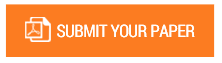Solving First Order Ordinary Differential Equations using Least Square Method: A comparative study

Authors : Parth Singh Pawar; Dhananjay R. Mishra; Pankaj Dumka

Volume/Issue : Volume 7 - 2022, Issue 3 - March

Scribd : https://bit.ly/37oG4mE

DOI : https://doi.org/10.5281/zenodo.6418458

In this research article an attempt has been made to examine the performance of Finite difference method (FDM) and Least square method (LSM) on the solution of first order ordinary differential equations (ODE). Both FDM and LSM are applied on the test problem and the results thus obtained are compared with the exact solution. It has been observed that for third degree basis function the results of LSM very close to the analytical result. On further increasing the degree the improvement in the result is very meagre and N=3 can be considered as the optimum solution for LSM. It has also been observed that the FDM is very sensitive to the number of grid points and deviates from the exact results by a substantive amount for lower number of nodes. Whereas the LSM is independent of the number of nodes.

Keywords : Least Square Method; Finite Difference Method; Ordinary Differential Equation; Python; Optimization.

#### CALL FOR PAPERS

Paper Submission Last Date
31 - December - 2023